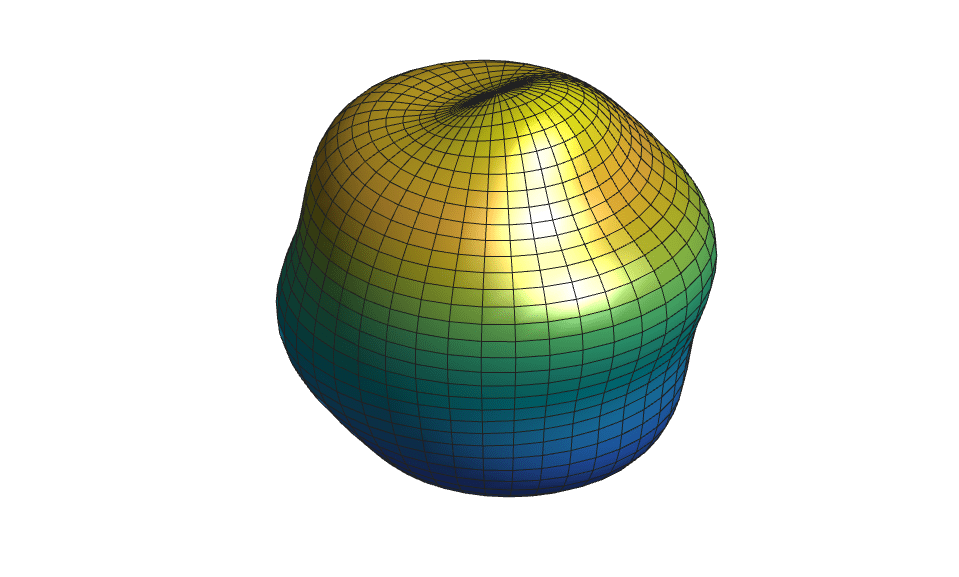# Animating a Surface

This example shows how to animate a surface. Specifically, this example animates a spherical harmonic. Spherical harmonics are spherical versions of Fourier series and can be used to model the free oscillations of the Earth.

### Define the Spherical Grid

Define a set of points on a spherical grid to calculate the harmonic.

```theta = 0:pi/40:pi; phi = 0:pi/20:2*pi; [phi,theta] = meshgrid(phi,theta); ```

### Calculate the Spherical Harmonic

Calculate the spherical harmonic with a degree of six, an order of one, and an amplitude of 0.5 on the surface of a sphere with a radius equal to five. Then, convert the values to Cartesian coordinates.

```degree = 6; order = 1; amplitude = 0.5; radius = 5; Ymn = legendre(degree,cos(theta(:,1))); Ymn = Ymn(order+1,:)'; yy = Ymn; for kk = 2: size(theta,1) yy = [yy Ymn]; end yy = yy.*cos(order*phi); order = max(max(abs(yy))); rho = radius + amplitude*yy/order; r = rho.*sin(theta); x = r.*cos(phi); y = r.*sin(phi); z = rho.*cos(theta); ```

### Plot the Spherical Harmonic on the Surface of a Sphere

Using the `surf` function, plot the spherical harmonic on the surface of the sphere.

```figure s = surf(x,y,z); light lighting gouraud axis equal off view(40,30) camzoom(1.5) ```### Animate the Surface

To animate the surface, use a for loop to change the data in your plot. To replace the surface data, set the `XData`, `YData`, and `ZData` properties of the surface to new values. To control the speed of the animation, use `pause` after updating the surface data.

```scale = [linspace(0,1,20) linspace(1,-1,40)]; for ii = 1:length(scale) rho = radius + scale(ii)*amplitude*yy/order; r = rho.*sin(theta); x = r.*cos(phi); y = r.*sin(phi); z = rho.*cos(theta); s.XData = x; s.YData = y; s.ZData = z; pause(0.05) end ```